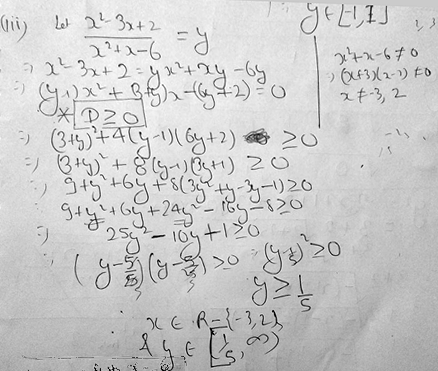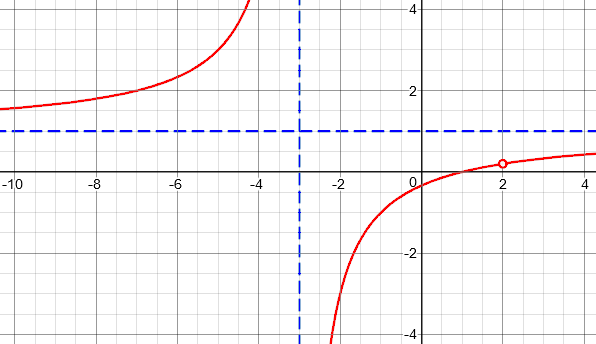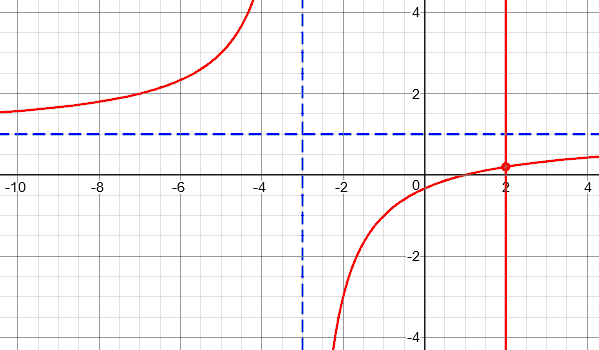# Finding the Range of a Tricky Rational Function

I previously wrote about finding the range of various kinds of functions. The examples there were relatively easy. A recent question raised the level of difficulty, bringing up some interesting issues.

Here is the initial question:

Hi,

I am trying to calculate the domain and range of this function f(x)= (x^2 – 3x + 2)/(x^2 + x – 6).The answer for range which I am getting is [1/5, infinity) but the correct answer given in my textbook is R-{1/5, 1}.

I will be thankful for help!

His notation for the correct answer means “all real numbers except 1/5 and 1”. His own answer is very different: all real numbers starting at 1/5.

First, let’s examine what he has done. He wants to find the range of $$f(x) = \frac{x^2 – 3x + 2}{x^2 + x – 6},$$ so he starts to solve the equation $$\frac{x^2 – 3x + 2}{x^2 + x – 6} = y,$$ by multiplying by the denominator and simplifying. The result is the quadratic equation $$(y-1)x^2 + (3+y)x – (6y + 2) = 0.$$ Solving this for x would give the inverse relation (x as a function of y); this technique is demonstrated in the previous post I mentioned above. We need to find the domain of this inverse relation, which will be the range of the original function. (That is, we’re finding the values of y for which an x exists.) In order to do that, we find the discriminant $$D = b^2 – 4ac$$; the range will be the set of values of y for which this is non-negative, so that there is at least one real value of x associated with that y. (As we’ll see, a little more than that will be needed.)

The result is an inequality that simplifies to $$25y^2 – 10y + 1 \gt 0.$$ But then he solves this inequality incorrectly …

I replied, pointing out this error (but making one of my own) and suggesting another way, by which I had obtained the correct answer:

One specific error in your work is the step from (y – 1/5)2 ≥ 0 to y ≥ 1/5. Polynomial inequalities don’t work that way; the solution in this case is y ≠ 1/5, because the square is positive unless the base is 0.

I solved this a different way; trying it your way led to some subtle steps I almost missed. I did get the correct answer both ways.

I first simplified the function by canceling (x – 2). Then I found the inverse and determined its domain (which could be done directly due to the simplification). I also had to eliminate the value of y corresponding to the “hole” in the original function.

My method started by factoring the numerator and denominator and simplifying:

$$f(x) = \frac{x^2 – 3x + 2}{x^2 + x – 6}$$

$$f(x) = \frac{(x-2)(x-1)}{(x-2)(x+3)}$$

$$f(x) = \frac{x-1}{x+3},\ x\ne 2$$

This is equivalent to the original, as long as we take note that 2 is not in the domain. (That is the “hole”.)

Solving for x, we have

$$\frac{x-1}{x+3} = y$$

$$x-1 = y(x+3)$$

$$x – 1 = yx + 3y$$

$$x(1 – y) = 3y + 1$$

$$x = \frac{3y + 1}{1 – y}$$

This is defined for all y except 1. But we also have to exclude the value of y for which $$x = 2$$ in this simplified equation, which is $$y = \frac{2-1}{2+3} = \frac{1}{5}.$$

The range is therefore, as the book said, all real numbers except 1 and 1/5.

But I had mentioned further subtleties if we don’t take this way, and the student chose to persevere in order to learn how to handle obstacles:

Thank You Doctor Peterson for help!

I am trying to proceed by my own method.

So, you are saying that if (y – 1/5)2 ≥ 0 then it can’t be  y ≥ 1/5, and y ≠ 1/5.

But, there is an equality symbol as well.

So, I think y = 1/5 is true. Why do you think it is false?

So, once again the range which I am getting is from 1/5 (included) to infinity.

But, the correct range given in my textbook is All Real except 1/5 and 1.

I will be thankful for help.

His correction of my correction is correct! I had treated the inequality as “>” instead of “≥”. But that only deepens the mystery: How is 1/5 excluded from the domain of the inverse? I gave some hints for extending his method to find the correct answer:

I did miss the equality part (probably because of the correct solution you’d mentioned); but I hope you see that the solution of (y – 1/5)2 ≥ 0 is not x ≥ 1/5, but all real numbers! A square is never negative.

So what is special about 1/5 that would exclude it from the domain? The discriminant there is 0, so there is only one solution for x; what is that one solution? Find out, and then look back at the original equation!

This is one of the subtleties I mentioned in your method; but it also occurred in mine, and I mentioned it in my summary of what I did.

Now, what about the exclusion of 1? That’s the other major subtlety: Look at your quadratic equation in x; is it always quadratic? If it isn’t, then the discriminant isn’t really relevant; you have to take this as a special case.

So far, his method has not yielded any numbers to be excluded; they will both be special cases, somehow. So figuring this out will be useful for understanding the whole process.

He replied:

Ok so I plugged y = 1/5 in the equation and got x = 2, which is of course rejected because x ≠ 2.

So, my final question is that how would someone decide which values to plug in the equation to check whether it is possible or not? (For example, how would someone decide that he/she need to check for 1/5 and not for any other number ?)

This is actually the easier of the two exclusions to deal with, but it turns out to be not really about checking y = 1/5, but rather checking x = 2:

I suppose the fact that this is a special point (where the discriminant is 0) suggests checking; but, really, from the start you should plan to check the value of y when x=2 (in the original equation, after removing the “hole”), and exclude that, as long as it isn’t associated with any other value of x. (That’s another subtle point.) So dealing with that point is really separate from the work you did.

Ultimately, I would want to sketch the graph along with any algebraic methods I use, to make sure I haven’t missed anything important (like that hole).

So, the correct way to find this really has nothing to do with finding 1/5 in the course of his work, but merely with his failure to even consider the hole! Because he never factored the numerator and denominator, he was unaware of the need to exclude the y associated with the hole.

But this does leave a curiosity: How did his method end up coming so close, with 1/5 as a special point at all?

Let’s look at his work, but with the numerator and denominator written in factored form:

$$\frac{(x-2)(x-1)}{(x-2)(x+3)} = y\ \Rightarrow\ (x-2)(x-1) = (x-2)(x+3)y.$$

We have the factor $$(x-2)$$ on both sides, so that when $$x = 2$$, the equation is true regardless of the value of y. In fact, we could therefore rewrite that equation as $$(x-2)\left((y-1)x + (3y+1)\right) = 0,$$ whose solutions are $$x = 2$$ and $$\displaystyle x = \frac{3y+1}{1-y}$$. So when the discriminant is 0 (so that there is one solution), these are equal, and $$\displaystyle\frac{3y+1}{1-y} = 2$$. This is, of course, when $$y = 1/5$$! So it just happens (!) that the check for the discriminant did locate the hole, and there really was reason to check that point. I doubt I would ever have guessed that.

Now let’s finally look at a graph of this function:We can see that the domain excludes -3 (the vertical asymptote) and 2 (the hole); and the range excludes 1 (the horizontal asymptote) and 1/5 (the hole).

(By the way, did you wonder at some point why the discriminant was almost always positive, indicating that there were two values of x for a given value of y? Did you assume that therefore the function must not be one-to-one? It’s because the equation we were working with there consisted of this graph together with the vertical line x = 2.)There’s still one thing missing, though: How would the student’s method have found that y can’t be 1 (the asymptote)? This is my second hint, which I haven’t yet elaborated. Look back at the student’s quadratic equation: $$(y-1)x^2 + (3+y)x – (6y + 2) = 0.$$ The discriminant only applies to quadratic equations; and this is not a quadratic equation when y = 1! So we should have checked that case before finding the discriminant. And this amounts to finding the horizontal asymptote. In my method, I actually found the inverse, so this asymptote was directly visible. In the method we are examining, we looked only at whether an inverse exists, and never actually saw it, so as to discover these details.

What’s the bottom line of all this? Don’t skip the factoring and simplifying when you work with a rational function!

### 2 thoughts on “Finding the Range of a Tricky Rational Function”

1.Find the range of (4x^2-36)/(x^2-2x-8).

1.This would be a good question to submit on our Ask a Question page, so we can discuss it together. I’ll ask you to show what you have tried, and what specific questions you have about it.

This particular problem doesn’t have the same issues as the one in this post; there are no “holes”, and the answer is very simple. If you use the discriminant, you will end up with a quadratic inequality that is not pretty, but has a simple solution. If you find the inverse, no factors will cancel as they did in my work.

But it may be well worth discussing your work, wherever you are having trouble.

This site uses Akismet to reduce spam. Learn how your comment data is processed.